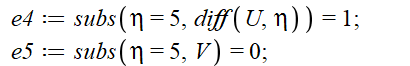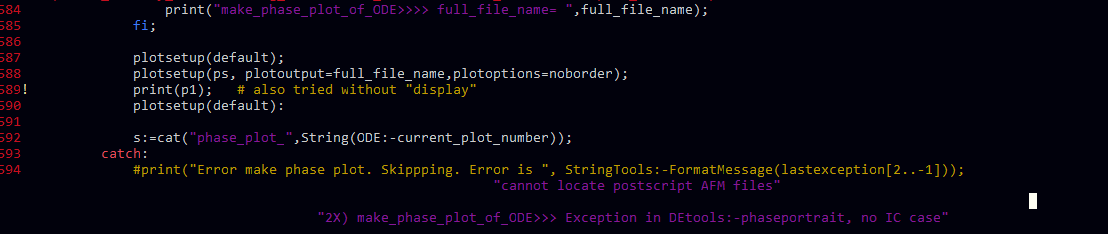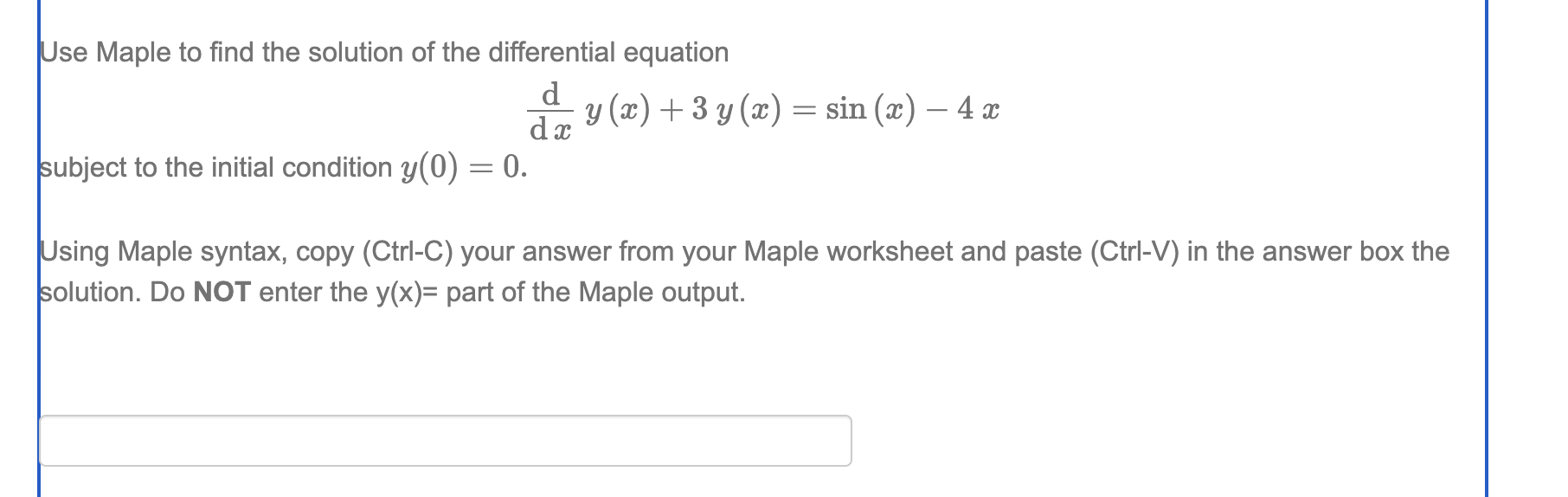## How to fix a curve...

Can you change f(eta) to upflow curve and theta(eta) to downflow curve.

In my Problem,Boundary Conditions are

theta(infinity) = 0, (D(f))(infinity) = 1 , (Take, eta =infinity)

Flows will be correct for what value is taken for infinity .

I take  eta = 5. and also tried changing ranges  but could't find it.Please Help to fix the curve.my code is,

SM.mw

## Can anyone integrate dsolve/numeric with NLPSolve ...

In a recent question/conversation, I had discussed integrating dsolve/numeric-based codes with NLPsolve at
https://www.mapleprimes.com/questions/236494-Inconsistent-Behavior-With-Dsolvenumeric-And-NLPSolve-sqp

@acer was able to help resolve the issue by calling NLPSolve with higher optimality tolerance.

I am starting a new question/post to show the need for evalhf as the previous post takes too long to load.
Code is attached for the same.

 > restart:
 > currentdir();Test code written by Dr. Venkat Subramanian at UT Austin, 05/31/2023 and has been updated multiple times. This code uses CVP approach (piecwise constant) to perform optimal control. NLPSolve combination with RK2 approach to integrate ODEs (constant step size) works well for this problem. But dsolve numeric based codes work (with optimality tolerance fix from acer), but cannot handle large values of nvar (optimization variables).

 > Digits:=15;>
 > eqodes:=[diff(ca(t),t)=-(u+u^2/2)*1.0*ca(t),diff(cb(t),t)=1.0*u*ca(t)-0.1*cb(t)];> soln:=dsolve({op(eqodes),ca(0)=alpha,cb(0)=beta},type=numeric,'parameters'=[alpha,beta,u],savebinary=true):
 >
 >

Note that Vector or Array form can be used for RK2h to implement the procedure for any number of variables/ODEs. But the challenge will be when implicit methods are used to integrate ODEs/DAEs and running them in evalhf form (this can be done with Gauss elimination type linear solver, but this will be limited to small number of ODEs/DAEs say 200 or so).

 > RK2h:=proc(NN,u,dt,y0::Vector(datatype=float))#this procedure can be made efficient in vector form local j,c1mid,c2mid,c10,c20,c1,c2; option hfloat; c10:=y0;c20:=y0; for j from 1 to NN do   c1mid:=c10+dt/NN*(-(u+u^2/2)*c10):   c2mid:=c20+dt/NN*(u*c10)-0.1*dt/NN*c20:   c1:=c10/2+c1mid/2+dt/NN/2*(-(u+u^2/2)*c1mid):   c2:=c20/2+c2mid/2+dt/NN/2*(u*c1mid)-0.1*dt/NN/2*c2mid:   c10:=c1:c20:=c2:   od: y0:=c10:y0:=c20: end proc:
 >
 > soln('parameters'=[1,0,0.1]);soln(0.1);>
 >
 > ssdsolve:=proc(x) interface(warnlevel=0): #if  type(x,Vector)#if type is not needed for this problem, might be needed for other problems #then local z1,n1,i,c10,c20,dt,u; global soln,nvar; dt:=evalf(1.0/nvar): c10:=1.0:c20:=0.0: for i from 1 to nvar do u:=x[i]: soln('parameters'=[c10,c20,u]): z1:=soln(dt): c10:=subs(z1,ca(t)):c20:=subs(z1,cb(t)): od: -c20;  #else 'procname'(args): #end if: end proc:
 >
 >
 > ssRK2:=proc(x)#based on RK2 #interface(warnlevel=0): option hfloat; #if  type(x,Vector) #then local z1,n1,i,c10,c20,dt,u,NN,y0; global nvar,RK2h; y0:=Array(1..2,[1.0,0.0],datatype=float): #y0:=1.0:y0:=0.0: dt:=evalf(1.0/nvar): NN:=256*2/nvar;#NN is hardcode based on the assumption that nvar will be a multiple of 2 <=256 for i from 1 to nvar do u:=x[i]: evalhf(RK2h(NN,u,dt,y0)): od: -y0;  #else 'procname'(args): #end if: end proc:
 > nvar:=2;> ic0:=Vector(nvar,[seq(0.1,i=1..nvar)],datatype=float):
 > bl := Vector(nvar,[seq(0.,i=1..nvar)],datatype=float):bu := Vector(nvar,[seq(5.,i=1..nvar)],datatype=float):
 > infolevel[Optimization]:=15;> CodeTools:-Usage(Optimization:-NLPSolve(nvar,evalhf(ssRK2),[],initialpoint=ic0,[bl,bu],optimalitytolerance=1e-6)):

NLPSolve: calling NLP solver

NLPSolve: using method=sqp

NLPSolve: number of problem variables 2

NLPSolve: number of nonlinear inequality constraints 0

NLPSolve: number of nonlinear equality constraints 0

NLPSolve: number of general linear constraints 0

NLPSolve: feasibility tolerance set to 0.1053671213e-7

NLPSolve: optimality tolerance set to 0.1e-5

NLPSolve: iteration limit set to 50

NLPSolve: infinite bound set to 0.10e21

NLPSolve: trying evalhf mode

NLPSolve: trying evalf mode

attemptsolution: number of major iterations taken 11

memory used=0.96MiB, alloc change=0 bytes, cpu time=16.00ms, real time=121.00ms, gc time=0ns

 >

Calling the procedures based on RK2 with NLPSolve uses evalf for numerical gradient (fdiff). Providing a procedure for gradient solves this issue.

 > gradRK2 := proc (x,g) option hfloat; local base, i, xnew, del; global nvar,ssRK2; xnew:=Array(1..nvar,datatype=float): base := ssRK2(x); for i to nvar do xnew[i] := x[i]; end do; for i to nvar do del := max(1e-5,.1e-6*x[i]); xnew[i] := xnew[i]+del; g[i] := (ssRK2(xnew)-base)/del; xnew[i] := xnew[i]-del; end do; end proc:

NLPSolve: calling NLP solver

NLPSolve: using method=sqp

NLPSolve: number of problem variables 2

NLPSolve: number of nonlinear inequality constraints 0

NLPSolve: number of nonlinear equality constraints 0

NLPSolve: number of general linear constraints 0

NLPSolve: feasibility tolerance set to 0.1053671213e-7

NLPSolve: optimality tolerance set to 0.1e-5

NLPSolve: iteration limit set to 50

NLPSolve: infinite bound set to 0.10e21

NLPSolve: trying evalhf mode

attemptsolution: number of major iterations taken 11

memory used=184.77KiB, alloc change=0 bytes, cpu time=31.00ms, real time=110.00ms, gc time=0ns

Significant saving in memory used is seen. CPU time is also less, which is more apparent at larger valeus of nvar.

 >

dsolvenumeric based codes work for optimization, but evalf is invoked possibly for both the objective and gradient

 > CodeTools:-Usage(Optimization:-NLPSolve(nvar,(ssdsolve),[],initialpoint=ic0,[bl,bu],optimalitytolerance=1e-6)):

NLPSolve: calling NLP solver

NLPSolve: using method=sqp

NLPSolve: number of problem variables 2

NLPSolve: number of nonlinear inequality constraints 0

NLPSolve: number of nonlinear equality constraints 0

NLPSolve: number of general linear constraints 0

NLPSolve: feasibility tolerance set to 0.1053671213e-7

NLPSolve: optimality tolerance set to 0.1e-5

NLPSolve: iteration limit set to 50

NLPSolve: infinite bound set to 0.10e21

NLPSolve: trying evalhf mode

NLPSolve: trying evalf mode

attemptsolution: number of major iterations taken 11

memory used=14.61MiB, alloc change=37.00MiB, cpu time=94.00ms, real time=213.00ms, gc time=46.88ms

 >

Providing gradient procedure doesn't help with evalhf computaiton for dsolve/numeric based procedures.

 > graddsolve := proc (x,g) local base, i, xnew, del; global nvar,ssdsolve; #xnew:=Vector(nvar,datatype=float): xnew:=Array(1..nvar,datatype=float): base := ssdsolve(x); for i to nvar do xnew[i] := x[i]; end do; for i to nvar do del := max(1e-5,.1e-6*x[i]); xnew[i] := xnew[i]+del; g[i] := (ssdsolve(xnew)-base)/del; xnew[i] := xnew[i]-del; end do; end proc:

NLPSolve: calling NLP solver

NLPSolve: using method=sqp

NLPSolve: number of problem variables 2

NLPSolve: number of nonlinear inequality constraints 0

NLPSolve: number of nonlinear equality constraints 0

NLPSolve: number of general linear constraints 0

NLPSolve: feasibility tolerance set to 0.1053671213e-7

NLPSolve: optimality tolerance set to 0.1e-5

NLPSolve: iteration limit set to 50

NLPSolve: infinite bound set to 0.10e21

NLPSolve: trying evalhf mode

NLPSolve: trying evalf mode

attemptsolution: number of major iterations taken 11

memory used=3.82MiB, alloc change=0 bytes, cpu time=31.00ms, real time=129.00ms, gc time=0ns

 >

Calling both RK2 and dsolve based procedures again to check the values and computation meterics

NLPSolve: calling NLP solver

NLPSolve: using method=sqp

NLPSolve: number of problem variables 2

NLPSolve: number of nonlinear inequality constraints 0

NLPSolve: number of nonlinear equality constraints 0

NLPSolve: number of general linear constraints 0

NLPSolve: feasibility tolerance set to 0.1053671213e-7

NLPSolve: optimality tolerance set to 0.1e-5

NLPSolve: iteration limit set to 50

NLPSolve: infinite bound set to 0.10e21

NLPSolve: trying evalhf mode

attemptsolution: number of major iterations taken 11

memory used=185.09KiB, alloc change=0 bytes, cpu time=16.00ms, real time=95.00ms, gc time=0ns

NLPSolve: calling NLP solver

NLPSolve: using method=sqp

NLPSolve: number of problem variables 2

NLPSolve: number of nonlinear inequality constraints 0

NLPSolve: number of nonlinear equality constraints 0

NLPSolve: number of general linear constraints 0

NLPSolve: feasibility tolerance set to 0.1053671213e-7

NLPSolve: optimality tolerance set to 0.1e-5

NLPSolve: iteration limit set to 50

NLPSolve: infinite bound set to 0.10e21

NLPSolve: trying evalhf mode

NLPSolve: trying evalf mode

attemptsolution: number of major iterations taken 11

memory used=3.82MiB, alloc change=0 bytes, cpu time=31.00ms, real time=122.00ms, gc time=0ns

 > s1RK2;s1dsolve;>

Next, a for loop is written to optimize for increasing values of nvar. One can see that evalhf is important for larger values of nvar. While dsolve/numeric is a superior code, not being able to use it in evalhf format is a significant weakness and should be addressed. Note that dsolve numeric evalutes procedures that are evaluated in evalhf or compiled form, so hopefully this is an easy fix.

 > infolevel[Optimization]:=0:
 > for j from 1 to 9 do nvar:=2^(j-1): ic0:=Vector(nvar,[seq(0.1,i=1..nvar)],datatype=float): bl := Vector(nvar,[seq(0.,i=1..nvar)],datatype=float):bu := Vector(nvar,[seq(5.,i=1..nvar)],datatype=float): soptRK[j]:=CodeTools:-Usage(Optimization:-NLPSolve(nvar,evalhf(ssRK2),[],initialpoint=ic0,[bl,bu],objectivegradient=gradRK2,optimalitytolerance=1e-6)): print(2^(j-1),soptRK[j]);
 > od:

memory used=88.40KiB, alloc change=0 bytes, cpu time=0ns, real time=10.00ms, gc time=0nsmemory used=155.66KiB, alloc change=0 bytes, cpu time=16.00ms, real time=30.00ms, gc time=0nsmemory used=175.36KiB, alloc change=0 bytes, cpu time=62.00ms, real time=80.00ms, gc time=0nsmemory used=243.69KiB, alloc change=0 bytes, cpu time=156.00ms, real time=239.00ms, gc time=0nsmemory used=260.09KiB, alloc change=0 bytes, cpu time=141.00ms, real time=260.00ms, gc time=0nsmemory used=385.84KiB, alloc change=0 bytes, cpu time=313.00ms, real time=545.00ms, gc time=0nsmemory used=0.65MiB, alloc change=0 bytes, cpu time=734.00ms, real time=1.18s, gc time=0nsmemory used=1.24MiB, alloc change=0 bytes, cpu time=1.55s, real time=2.40s, gc time=0nsmemory used=2.99MiB, alloc change=0 bytes, cpu time=3.45s, real time=5.92s, gc time=0ns> for j from 1 to 6 do nvar:=2^(j-1): ic0:=Vector(nvar,[seq(0.1,i=1..nvar)],datatype=float): bl := Vector(nvar,[seq(0.,i=1..nvar)],datatype=float):bu := Vector(nvar,[seq(5.,i=1..nvar)],datatype=float): soptdsolve[j]:=CodeTools:-Usage(Optimization:-NLPSolve(nvar,evalf(ssdsolve),[],initialpoint=ic0,[bl,bu],objectivegradient=graddsolve,optimalitytolerance=1e-6)): print(2^(j-1),soptdsolve[j]);
 > od:

memory used=0.66MiB, alloc change=0 bytes, cpu time=16.00ms, real time=11.00ms, gc time=0nsmemory used=3.79MiB, alloc change=0 bytes, cpu time=15.00ms, real time=52.00ms, gc time=0nsmemory used=21.28MiB, alloc change=0 bytes, cpu time=78.00ms, real time=236.00ms, gc time=0nsmemory used=144.82MiB, alloc change=-4.00MiB, cpu time=469.00ms, real time=1.60s, gc time=46.88msmemory used=347.30MiB, alloc change=16.00MiB, cpu time=1.27s, real time=3.45s, gc time=140.62msmemory used=1.33GiB, alloc change=-8.00MiB, cpu time=7.66s, real time=15.92s, gc time=750.00ms>
 > SS:=[seq(soptRK[j],j=1..9)];>
 > E1:=[seq(SS[i]-SS[i+1],i=1..nops(SS)-1)];To get 6 Digits accuracy we need nvar = 256 which may not be attainable with dsolve/numeric approach unless we are able to call it evalhf format.

 >
 >

## Error, invalid input: diff expects 2 or more argum...

Maple code for the problem is

TFBE.mw

## "cannot locate postscript AFM files" Shows only wh...

When running "C:\Program Files\Maple 2023\bin.X86_64_WINDOWS\cmaple.exe" on my application which makes plots and export them to ps, sometimes I get an exception

"cannot locate postscript AFM files"

This happens on some plots. Not all. The strange thing, using the same exact run, but from the worksheet, it works fine and I get the .ps generated with no such error. This only happens when running from the command line. It happens here

```
try
plotsetup(ps, plotoutput="plot.ps",plotoptions=noborder);
print(p1);
plotsetup(default):
catch:
print(StringTools:-FormatMessage( lastexception[2..-1] ));
end try;

```

where p1 above is a plot that was generated earlier OK with no error. I made sure there is no font settings on the plot or any extra options that might cause a problem.

I am not able to make MWE as this problem only shows deep inside my application and only when calling Maple from command line and only shows up on very few plots for some reason I do not understand.  When I made a small .mpl with same code used to make the plot, and run it from command line, the error do not show up. It only shows up when running the whole application from the command line.

My question is: How does Maple find these postscript AFM files? It looks like the postscript driver used by command like Maple is older than the one used by the frontend/GUI and this is why this error only shows from the command line. Has anyone seen such a Maple error before?

I have no idea where to find these AFM postscript files it says it can't find and what to do now. I am on windows 10.

Any suggestion what to lookfor and anything else I can do to help me find the cause of this?

If it is any help, here is the MWE I tried to reproduce this with. But this generated no error. Call this foo.mpl

```foo:=proc()
local p1,ode,y,x;
ode:=3*y(x)+x*diff(y(x),x) = 2*x^5;
p1 := DEtools:-phaseportrait(ode,y(x),x=-1..5,[y(2)=1],y=-1000..1000):
try
plotsetup(ps, plotoutput=cat("C:/tmp/plot.ps"),plotoptions=noborder);
print(p1);   # also tried without "display"
plotsetup(default):
catch:
print(StringTools:-FormatMessage( lastexception[2..-1] ));
print("Exception");
end try;
end proc;

foo();```

Then run

C:\tmp>"C:\Program Files\Maple 2023\bin.X86_64_WINDOWS\cmaple.exe"  foo.mpl

No error. The error only shows in my large application.

"cannot locate postscript AFM files"

Will keep trying to make a MWE, but for 3 hrs now, no luck yet.

Update

```if lasterrorlocus<>'lasterrorlocus' then
print(lasterrorlocus);
showsource();
end if; ```

Inside the catch, which print the exact location of the exception generated. It is at print(p1) as you seeagain, same code runs with no problem from the worksheet. This only shows when running from command line. Will keep trying to make MWE...

## how do I solve this one?...## Will Maple factorize certain HUGE polynomials (mod...

```(* taken from the links above *)